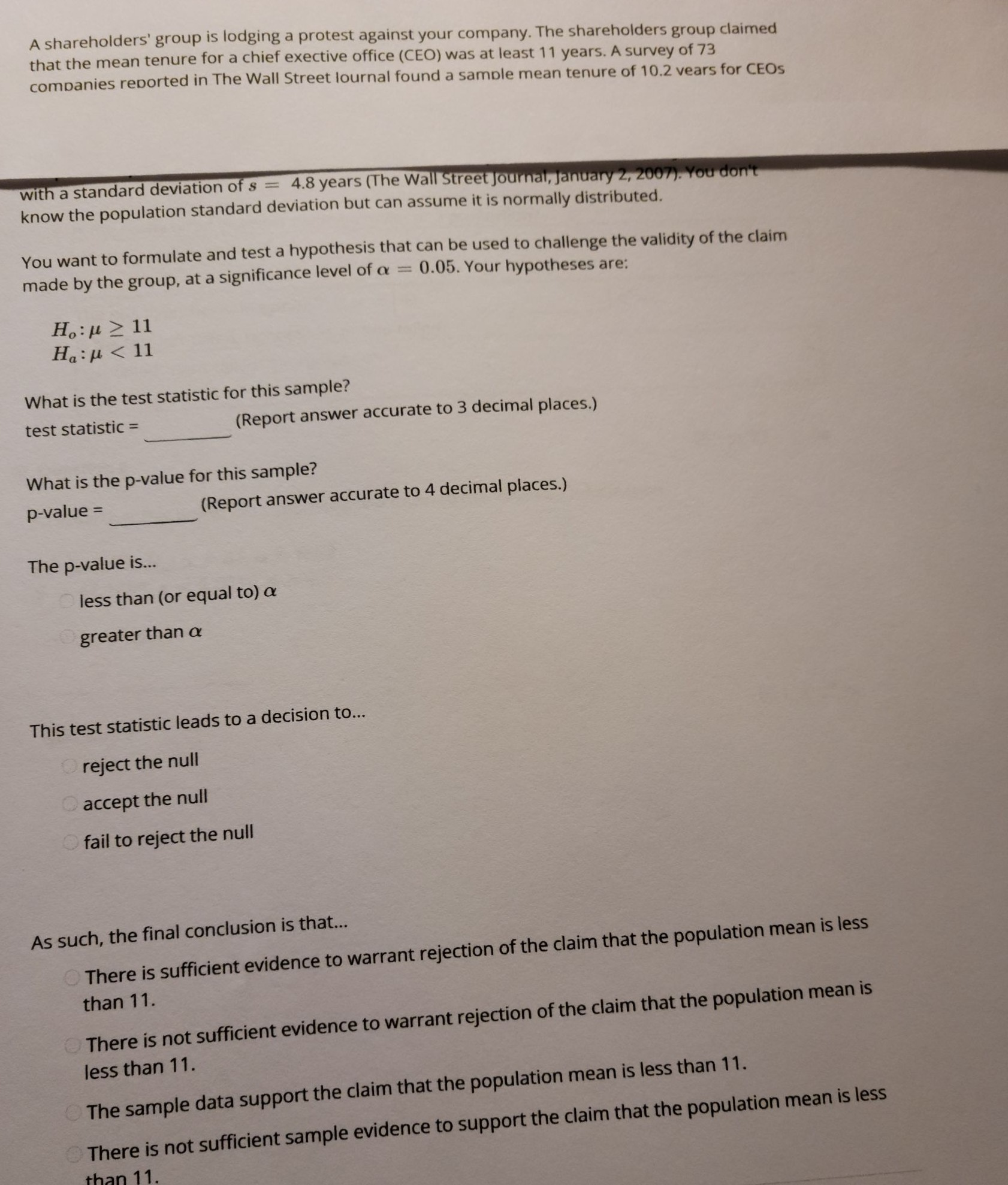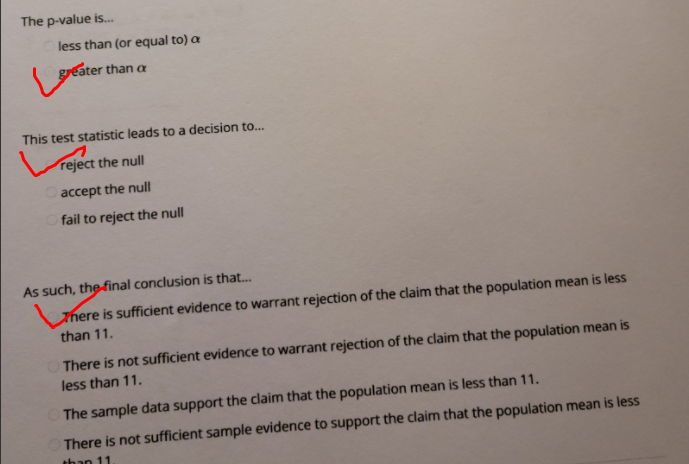### Still have math questions?

Algebra
QuestionA shareholders' group is lodging a protest against your company. The shareholders group claimed that the mean tenure for a chief exective office (CEO) was at least $$11$$ years. A survey of $$73$$ ammanies renorted in The Wall Street lournal found a samble mean tenure of $$10.2$$ vears for CEOs With a standard deviation of $$s = 4.8$$ years (The Wall Street joumal, january 2, $$2007$$ ). You don't know the population standard deviation but can assume it is normally distributed. You want to formulate and test a hypothesis that can be used to challenge the validity of the claim made by the group, at a significance level of $$\alpha = 0.05$$ . Your hypotheses are:

$$H _ { o } : \mu \geq 11$$

$$H _ { a } : \mu < 11$$

What is the test statistic for this sample?

test statistic $$=$$ (Report answer accurate to $$3$$ decimal places.)

What is the p-value for this sample? (Repalue $$=$$ The p-value is... less thanswer accurate to $$4$$ decimal places.) greater than $$\alpha$$

This test statistic leads to a decision to... reject the null

As such, the final conclusion is that... There is sufficient evidence to warrant rejection of the claim that the population mean is less than $$11 .$$ There is not sufficient evidence to warrant rejection of the claim that the population mean is less than $$11 .$$ The sample data support the claim that the population mean is less than $$11 .$$ There is not sufficient sample evidence to support the claim that the population mean is less than $$11$$ .